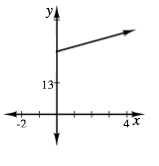### Home > A2C > Chapter 1 > Lesson 1.2.2 > Problem1-92

1-92.

Carlo got a pet snake as a birthday present. On his birthday, the baby snake was just $26$ cm long. He has been watching it closely and has noticed that it has been growing $2$ cm each week.

1. Create multiple representations ($x→y$ table, graph, and equation) of the function for which the inputs are the weeks since Carlo’s birthday and the outputs are the length of the snake.

Refer to problem 1-47.

2. If the snake continues to grow at the same rate, when will it be $1$ meter ($100$ cm) long? How can you see this in each representation?

What point represents the length of $100$ cm in each representation?
Show how to find the point using your graph, table, and equation.Create a representation by completing the table in the eTool below.
Click the link at right for the full version of the eTool:  1-92 HW eTool